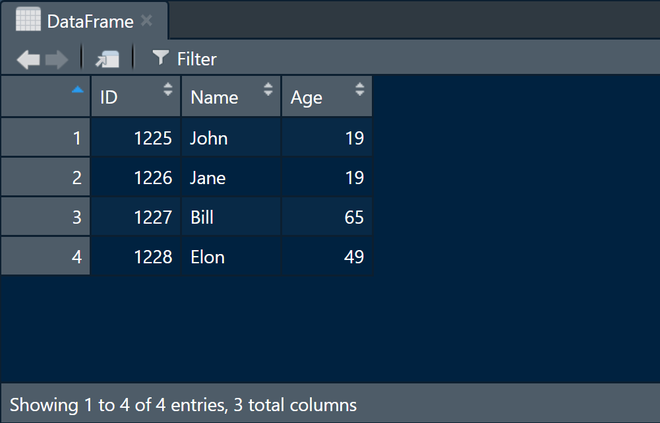Skip to content
Related Articles
How to convert excel content into DataFrame in R ?
• Last Updated : 17 Apr, 2021

R Programming Language allows us to read and write data into various files like CSV, Excel, XML, etc. In this article, we are going to discuss how to convert excel content into DataFrame in R Programming. To read an excel file itself, read.xlsx() function from xlsx is used.

### Installation

This module is not built into R but it can be installed and imported explicitly.  Open the R console and type the command given below to install xlsx package.

install.packages(“xlsx”)

read_xlsx() can be used in the following way:

Syntax: read.xlsx(“Excel File Path”, sheetName = “sheet name”, …)

Parameters: it is necessary to give the Excel file path and sheetName as argument in read.xlsx() function but many other parameters can be used by this function such as colNames, rowNames, skipEmptyRows, skipEmptyCols, rows, cols, etc. for doing some extra modifications. Here we used colIndex as parameter for getting only column that we want. here we give 1 as a value of colIndex parameter for getting first column of Excel file.

File in use:

First, we import xlsx package by using the library() function then give the full path of the Excel file to excel_path named variable. To create a dataframe keep extracting columns from the file and combine them into one data frame once done.

Program:

## R

 `library``(xlsx)`` ` ` ` `excel_path <- ``"excelContent.xlsx"`` ` `id <- ``read.xlsx``(excel_path, sheetName = ``"ageData"``, colIndex = 1)``Name <- ``read.xlsx``(excel_path, sheetName = ``"ageData"``, colIndex = 2)``Age <- ``read.xlsx``(excel_path, sheetName = ``"ageData"``, colIndex = 3)`` ` `DataFrame <- ``data.frame``(id, Name, Age)`

Output:DataFrame created from excel content

My Personal Notes arrow_drop_up